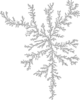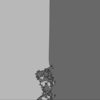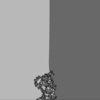# Pictures¶

Here are some pictures I produced of various random two-dimensional objects. In each case there is a small preview, and the full-resolution image itself is linked in case you would like to download it (some of the files can be quite large!). There is a discussion about some of the simulations themselves on this page and if I get the motivation someday I might set up separate pages for each model.

“Terms of use:” You can use any one of the images on this page in any way you see fit; just let me know when you do so.

One last thing: If you need pictures with a higher definition (or pictures of something else that I did not put here but might have simulated already), just ask!A DLA cluster of size 2000A DLA cluster of size 4096A DLA cluster of size 8192An Ising configuration (beta=0.881374)An Ising configuration (beta=0.900000)A loop-erased walk of size 2000Broken line processBond-percolation cluster (p=0.500000)Site-percolation cluster (p=0.580000)Gradient percolation clusterA pivoting point of angle pi/2A self-avoiding walk of length 10000Schramm’s SLE Process (kappa=1.000000)Schramm’s SLE Process (kappa=1.000000)Schramm’s SLE Process (kappa=2.000000)Schramm’s SLE Process (kappa=2.000000)Schramm’s SLE Process (kappa=3.000000)Schramm’s SLE Process (kappa=3.000000)Schramm’s SLE Process (kappa=3.500000)Schramm’s SLE Process (kappa=4.000000)Schramm’s SLE Process (kappa=4.000000)Schramm’s SLE Process (kappa=4.500000)Schramm’s SLE Process (kappa=5.000000)Schramm’s SLE Process (kappa=5.000000)Schramm’s SLE Process (kappa=6.000000)Schramm’s SLE Process (kappa=6.000000)Schramm’s SLE Process (kappa=6.000000)Schramm’s SLE Process (kappa=7.000000)Schramm’s SLE Process (kappa=7.000000)Schramm’s SLE Process (kappa=8.000000)Schramm’s SLE Process (kappa=8.000000)